A vessel at berth experiences much lower forces compared to a vessel in the open sea due to the milder environment, but it still requires a mooring configuration suited to the forces it experiences, and also suitable for the type of berthing configuration adopted. The berthing configuration depends on the geometry of the berth, and also on the available space depending on the traffic at the berth and size of the vessel. The most widely used berthing configuration is one with the vessel’s Port or Starboard side aligned with the Quay.

The configuration is shown in pictures below: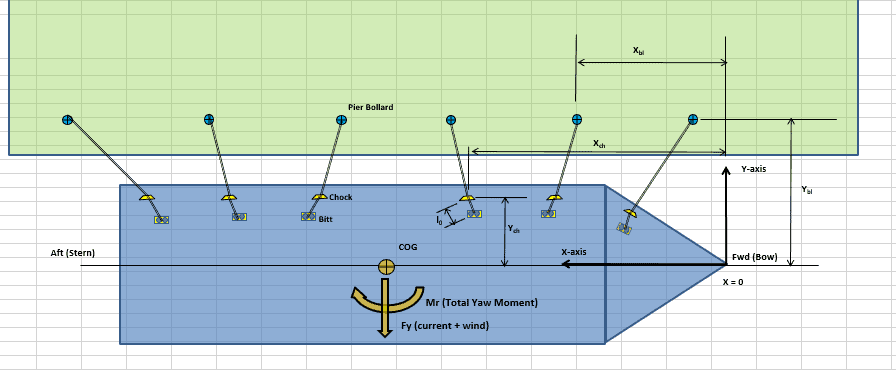A possible berth mooring arrangement

## Concept

For any configuration of mooring adopted, the design has to be adequate to restrain the forces which the vessel experiences. In this article, we will analyze the configuration with Port or Starboard on Quay in more detail, and present a simplified method for calculating the line loads for such a mooring pattern.

Thinking in very basic terms, the vessel will have its Port or Starboard pressing against the Quay (with fenders between the vessel and Quay to take the load). This vessel will be subjected to environmental loads coming from the fwd/aft end (longitudinal) and beam side of the vessel (athwartship) which will tend to drift it off away from the berth. A simple way is to tie the vessel to the quay side using ropes, and there could be many ways and configurations to do it.

We’ll be following a guideline developed by US Navy called DDS-582 to explain the method. Though DDS-582 has now been superseded by another document Mil-HDBK-1026_4a_Mooring Design, the principles outlined in DDS-582 are still effective and a good source of learning.

The next step will be to find out what line size and strength will be required to moor a vessel. The entire concept can be delineated in the following steps:

## Calculation Steps

• Get the vessel’s dimensions and cargo dimensions
• Get the environmental parameters of the Quay – wind speed and current speed (waves have been ignored)

• Calculate all the environmental forces on the vessel by using standard physical formulae and coefficients provided in DDS-582
• Calculate the line forces on the mooring lines by calculating the vessel displacement and mooring line stiffness, and using the spring formula F = kδ, where k is the mooring line stiffness and δ is the displacement of the vessel.

## Environmental Forces

When calculating the wind and current forces, the standard physics formula of Force = Pressure x Area shall be used. In the method by DDS-582, wave forces have not been considered for doing the calculation.

For wind force, the formula is Fwind = 1/2 x Cwind x ρwind x Vwind2 x Awind, where Cwind is the coefficient of wind force, ρwind is the density of air, Vwind is the wind speed, and Awind is the total area of ship and cargo which is exposed to the wind from head or beam direction (the above-water area)

Similarly, for current force, the formula is Fcurrent = 1/2 x Ccurrent x ρwater x Vcurrent2 x Aunderwater, where Ccurrent is the coefficient of current force, ρwater is the density of water, Vcurrent is the current speed, and Aunderwater is the total underwater area of ship which is exposed to the current from head or beam direction.

The forces need to be calculated for both the directions: head wind and beam wind.

The critical items in the calculations for Wind and Current forces are the force coefficients for wind and current.

These coefficients are obtained from the coefficient plots provided in DDS-582.

Coefficients are to be obtained for both longitudinal and transverse direction, and some plots are presented below: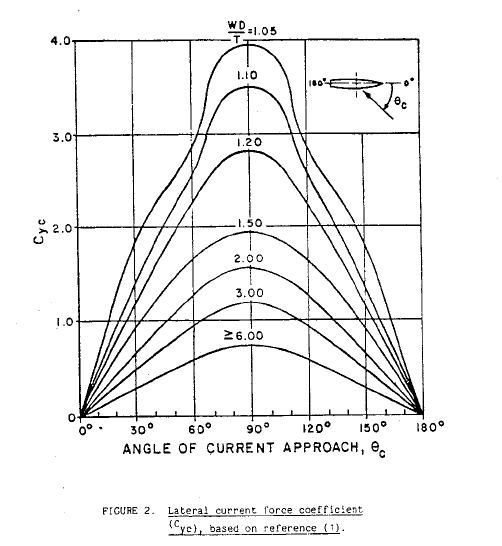Lateral Current Coefficient (DDS-582)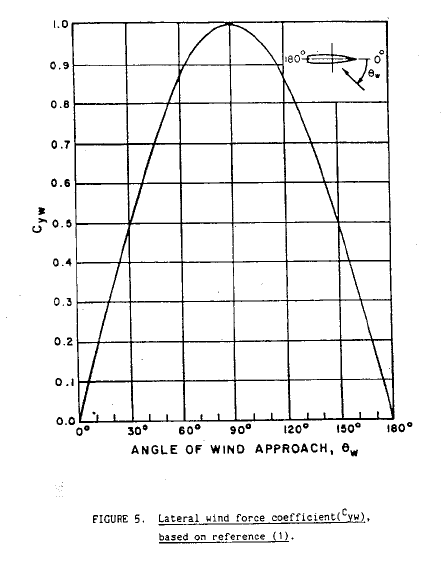Lateral Wind Coefficient (DDS-582)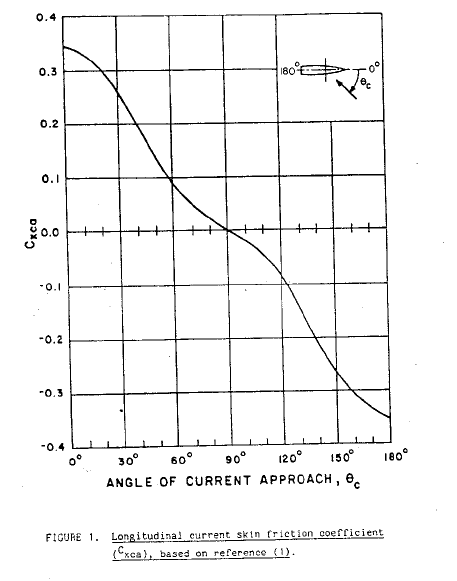Logitudinal Current Coefficient (DDS-582)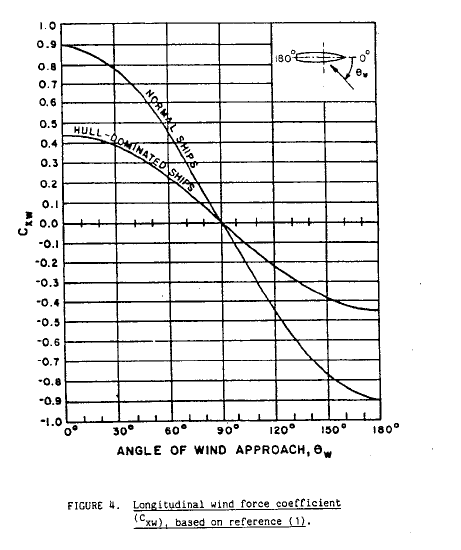Longitudinal Wind Coefficient (DDS-582)

Current Yaw Coefficient (DDS-582)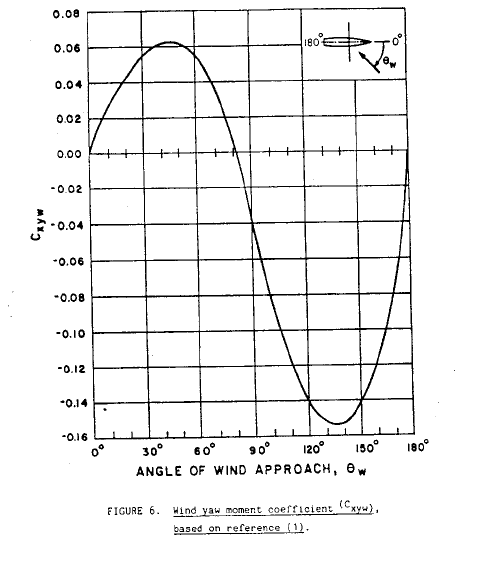Wind Yaw Coefficient (DDS-582)

We can see that the lateral current force and current yaw moment are dependent on an additional factor: the underkeel clearance which is measured as Water Depth to Draft ratio (WD/T).

Also, the longitudinal wind force coefficient is different for normal ships and hull-dominated ships. A hull dominated ship is one with minimum superstructure.

At the end of this exercise, we’ll have the following with us:

• Wind Force – longitudinal and transverse
• Wind Yaw moment
• Current force – longitudinal and transverse
• Current yaw moment

Next exercise is using these forces and calculate the line forces on individual mooring lines considering a quasi-static analysis, i.e., forces are assumed to be constant and not varying with time and dynamic nature of forces is neglected.

Line Force Calculation

Once the forces have been calculated, the calculation of the line forces is done by calculating the individual line stiffness and displacement. The pre-requisite for this is to have the mooring arrangement plan of the vessel prepared.

The mooring arrangement of the vessel depends on the location of mooring equipment on the vessel (i.e., location of mooring bollards/bitts/fairleads on the vessel) and also on the location of mooring equipment on the quayside.

Vessel owner/operator may like to try out different mooring configurations for the vessel’s berthing. Some guidelines for selecting the right mooring equipment are provided in DDS-582, and are also shown below: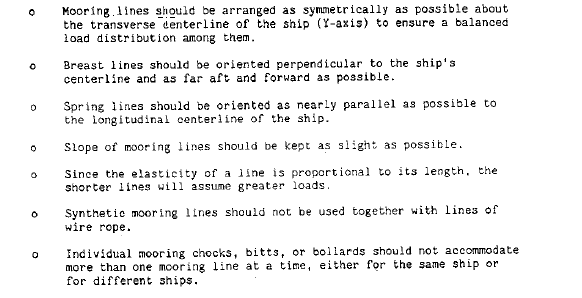Guidelines for Mooring Arrangement at berth

For any configuration of mooring, the principles of calculating the line tensions remains the same, and are lines out below:

1. From the mooring arrangement, calculate the geometric properties of the mooring lines, like their length, geometric projections in the horizontal and vertical planes etc.
2. Use each mooring line’s material and physical properties like Maximum Breaking Strength, section area, length etc. to calculate each line’s Stiffness

The stiffness in each direction (longitudinal, transverse, vertical) needs to be calculated

1. Use the external forces (see the section ‘Environmental Forces’ above) along with the stiffness values of all lines calculated in Step 2 to obtain the two displacement values of the vessel
1. Translation of ship in transverse direction (δY)
2. Rotation of the ship about vertical axis (γ)
2. Use the individual line stiffness and the displacements obtained above to calculate the individual line forces and its corresponding Factor of Safety

Factor of safety = Breaking Strength of line/Line force

The line forces calculated can be further used to select the right mooring equipment for the vessel.

The steps are detailed in DDS-582, and based on these a spreadsheet solution can be prepared. TheNavalArch has its own solution for mooring at berth based on DDS-582. You may like to check it out from the link below.